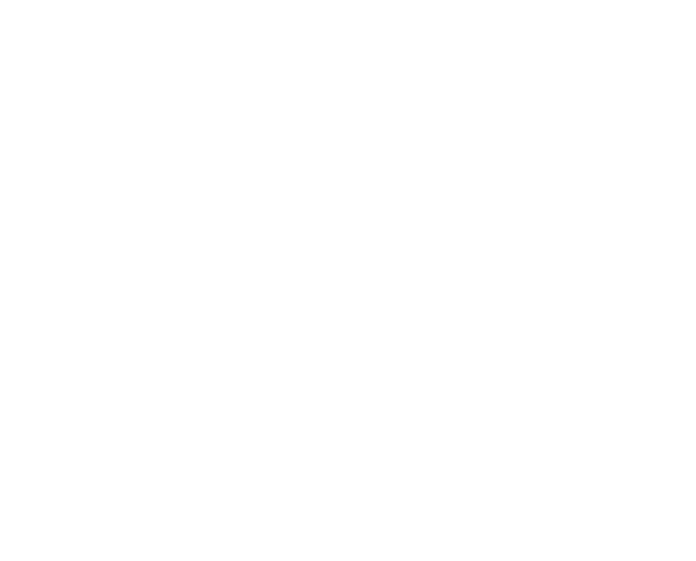Home Academic Leap Year in C, C for beginners

# Leap Year in C, C for beginners

0According to the definition, a year that is divisible by 4, 100 and 400 is known as a leap year. Also if a year is exactly divisible by 4 but not by 100 then it is also a leap year. Another important thing you must note is, if a year is exactly divisible by 4 and 100 but not by 400 then it is not a leap year. Based on this general understanding, we’ll build a programming logic to determine if a year entered by the user is leap year or not. We’ll also discuss how to print leap years between some range in this article.

## 1. Program to check whether a year entered by the user is leap year or not in C.

``````#include<stdio.h>
void main()
{
int yr;
printf("Enter year: ");
scanf("%d",&yr);
if(yr % 4 == 0)
{
if( yr % 100 == 0)
{
if ( yr % 400 == 0)
printf("%d is a Leap Year", yr);
else
printf("%d is not a Leap Year", yr);
}
else
printf("%d is a Leap Year", yr );
}
else
printf("%d is not a Leap Year", yr);
getch(); // for MS DOS based compilers like Turbo C
}``````

## 2. To print leap years in-between range of years provided by the user in C.

``````#include <stdio.h>
int main()
{
int year1, year2, i;
printf("Enter the Starting year: ");
scanf("%d", &year1);
printf("Enter the Ending Year: ");
scanf("%d", &year2);
printf("Leap Year between %d and %d are as below:\n",year1,year2);
for(i=Year1; i <= year2; i++){
if( (0 == i % 4) && (0 != i % 100) || (0 == i % 400) ){
printf("%d\n", i);
}
}
getch(); // for MS-DOS based compilers like Turbo C
}``````

Here, in the code portion

``````for(i=Year1; i <= year2; i++){
if( (0 == i % 4) && (0 != i % 100) || (0 == i % 400) ){
printf("%d\n", i);
}
}``````

for each ith year starting from starting year entered by user(i.e. initial value of i) as year1, we need to check if the year i is leap year or not? If yes, print it and if not keep iterating around loop until and unless value of i become ending year.

Thank You!

You may also check my previous articles